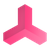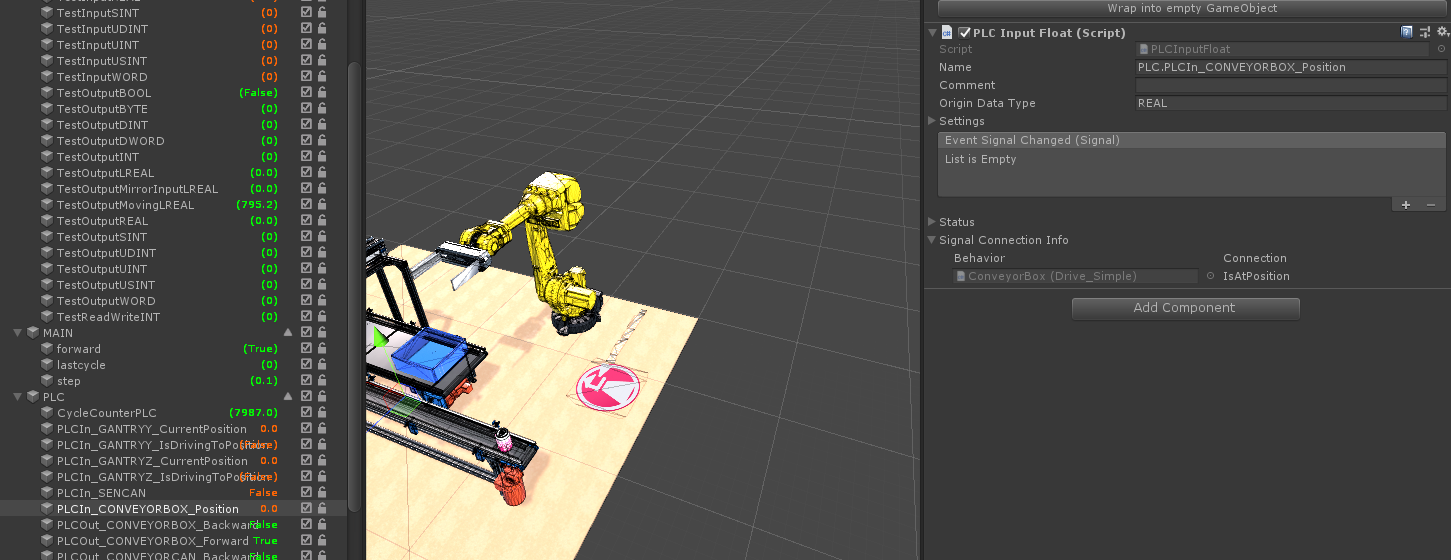0

# Writing to PLC inputs

leevi parssinen 4 years ago in realvirtual.io Starter and Professional updated by Support 4 years ago

Hi,

If i have PLC connected and imported signals...how would i approach writing for example a velocity of a rigid body into the value of a PLC signal under TwinCATInterface?Under review

Hi Leve,

I assume that you are having PLCInputs and PLCOutputs in your project after importing from TwinCAT:Now you can write to the PLCInput with

PLCInput.Value = YourValue;

You should write your own behavior scripts and attach this to the Gameobject with the rigid-body where you want to measure the velocity.

Here is a simple example of a behavior script (it is the Sensor_standard script from the Game4Auomation Framework).

This script is sending a "High" to the PLC when the sensor is occupied.

`namespace game4automation{    [RequireComponent(typeof(Sensor))]    //! The Sensor_Standard component is providing the Sensor behavior and connection to the PLC inputs and outputs.    public class Sensor_Standard : BehaviorInterface    {               [Header("Settings")] public bool NormallyClosed = false;  //!< Defines if sensor signal is *true* if occupied (*NormallyClosed=false*) of if signal is *false* if occupied (*NormallyClosed=true*)        [Header("Interface Connection")] public PLCInputBool Occupied; //! Boolean PLC input for the Sensor signal.        private Sensor Sensor;        private bool _isOccupiedNotNull;        // Use this for initialization        void Start()        {            _isOccupiedNotNull = Occupied != null;            Sensor = GetComponent<Sensor>();        }        // Update is called once per frame        void Update()        {            bool occupied = false;                    // Set Behavior Outputs            if (NormallyClosed)            {                occupied = !Sensor.Occupied;            }            else            {                occupied = Sensor.Occupied;            }            // Set external PLC Outputs            if (_isOccupiedNotNull)                Occupied.Value = occupied;        }    }}    `GOOD, I'M SATISFIED
Satisfaction mark by leevi parssinen 4 years agoUnder review

Hi Leve,

I assume that you are having PLCInputs and PLCOutputs in your project after importing from TwinCAT:Now you can write to the PLCInput with

PLCInput.Value = YourValue;

You should write your own behavior scripts and attach this to the Gameobject with the rigid-body where you want to measure the velocity.

Here is a simple example of a behavior script (it is the Sensor_standard script from the Game4Auomation Framework).

This script is sending a "High" to the PLC when the sensor is occupied.

`namespace game4automation{    [RequireComponent(typeof(Sensor))]    //! The Sensor_Standard component is providing the Sensor behavior and connection to the PLC inputs and outputs.    public class Sensor_Standard : BehaviorInterface    {               [Header("Settings")] public bool NormallyClosed = false;  //!< Defines if sensor signal is *true* if occupied (*NormallyClosed=false*) of if signal is *false* if occupied (*NormallyClosed=true*)        [Header("Interface Connection")] public PLCInputBool Occupied; //! Boolean PLC input for the Sensor signal.        private Sensor Sensor;        private bool _isOccupiedNotNull;        // Use this for initialization        void Start()        {            _isOccupiedNotNull = Occupied != null;            Sensor = GetComponent<Sensor>();        }        // Update is called once per frame        void Update()        {            bool occupied = false;                    // Set Behavior Outputs            if (NormallyClosed)            {                occupied = !Sensor.Occupied;            }            else            {                occupied = Sensor.Occupied;            }            // Set external PLC Outputs            if (_isOccupiedNotNull)                Occupied.Value = occupied;        }    }}    `